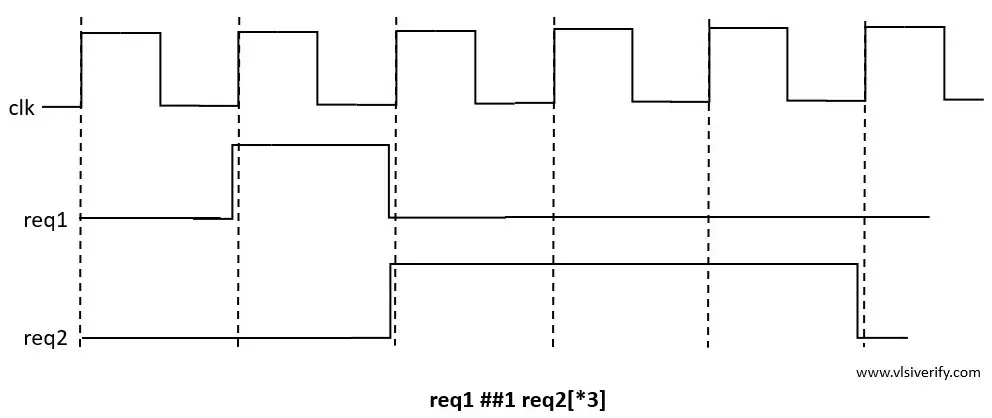Tutorials

## Clock delays

1. ##  : represents cycle delay

2. ##n – represents “n” clock cycles

3. ##0 – represents same clock cycle

4. ## [min:max] represents a range of clock cycles. Where min and max must be 0 or greater than 0.

``````sequence seq;
@(posedge clk) req1 ## [2:6] req2;
endsequence``````

The sequence will be matched if req1 is true then within clock cycle delay of range 2 to 6, req2 should be high.

5. \$ represents an infinite number of clock cycles i.e. till the end of the simulation.

``````sequence seq;
@(posedge clk) req1 ## [2:\$] req2;
endsequence``````

The sequence will be matched if req1 is true then req2 can be high at any time before simulation ends.

## Repetition Operator

If the sequence of events happens repeatedly for n times and it is represented as [*n] where “n” > 0 and “n” can not be \$.

Example 1:

``````sequence seq;
@(posedge clk) req1 ##1 req2[*3];
endsequence``````In this example, if req1 is true then after 1 clock cycle, req2 must be true for 3 consecutive clock cycles.

``req1 ##1 req2[*3] is same as req1 ##1 req2 ##1 req2 ##1 req2.``

Example 2:

The repetition operator can also be used in a certain range using [*m:n] where both “m” and “n” > 0 and n can not be \$.

``````sequence seq;
@(posedge clk) req1 ##1 req2[*2:4];
endsequence``````

In this example, if req1 is true then after 1 clock cycle, req2 must be true for a minimum of 2 and a maximum of 4  consecutive clock cycles.

``````req1 ##1 req2[*2:4] is same as
req1 ##1 req2 ##1 req2 or
req1 ##1 req2 ##1 req2 ##1 req2 or
req1 ##1 req2 ##1 req2 ##1 req2 ##1 req2;``````

## Non-consecutive repetitive operator

If sequence event repetition has to be detected for n non-consecutive clock cycles then [=n] can be used where n > 0 and n can not be \$.

``````sequence seq;
@(posedge clk) req1 ##1 req2[=4];
endsequence``````

In the above example, once req1 holds true after one clock cycle req2 must be true for 4 clock cycles but it is not mandatory to be consecutive clock cycles.

## The non-consecutive repetitive operator with a range

The non-consecutive repetition can be mentioned in a range as [=m:n] where minimum “m” and maximum “n” non-consecutive repetition.

``````sequence seq;
@(posedge clk) req1 ##1 req2[=2:4];
endsequence``````

In the above example, once req1 holds true after one clock cycle, req2 must be true for a minimum of 2 and a maximum of 4 clock cycles but it is not mandatory to be consecutive clock cycles.

SystemVerilog Assertions